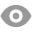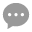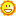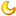# 唐奇安趋势系统测试和代码汇总240 |9 |王博 | 2020-4-26 11:31:27 | 显示全部楼层 |阅读模式
 唐奇安趋势系统定义与测试 1）定义唐奇安做多系统： 文字描述1：25日移动平均线与350日移动平均线呈金叉状态 量化定义：EMA25>EMA350 文字描述2：最高价突破20日新高 量化定义：当日最高价=近20日（含当日）最高价 文字描述3：最低价突破10日新低 量化定义：当日最低价=近10日（含当日）最低价 文字描述4：收盘价低于买入价格2倍ATR时，止损 量化定义：收盘价<=买入价格-2*ATR时，止损 定义测试的买卖逻辑：测试的买入条件：若25日均线在350日均线上方，并且最高价突破20日新高，以当日收盘价买入测试的平仓条件：若最低价突破10日新低或收盘价低于买入价格2倍ATR，以当日收盘价平仓 2）定义唐奇安做空系统： 文字描述1：25日移动平均线与350日移动平均线呈死叉状态 量化定义：EMA25=买入价格+2*ATR时，止损 定义测试的买卖逻辑：测试的买入条件：若25日均线在350日均线下方，并且最低价突破20日新低，以当日收盘价买入测试的平仓条件：若最高价突破10日新高或收盘价高于买入价格2倍ATR时，以当日收盘价平仓（相关阅读：《唐奇安趋势系统炒股原理，唐奇安趋势系统指标源码止损公式》）

 测试一：(1) 唐奇安做多系统 【测试代码】//定义仓位FUND:=10000000;LOTS:=INTPART(FUND*0.8/(CLOSE+FEE));//计算手数，使每次开仓金额都是800W //定义ATRTR:=MAX(MAX((H-L),ABS(REF(C,1)-H)),ABS(REF(C,1)-L));//求最高价减去最低价，一个周期前的收盘价减去最高价的绝对值，一个周期前的收盘价减去最低价的绝对值，这三个值中的最大值ATR:=MA(TR,26);//求N个周期内的TR的简单移动平均 //定义指数移动平均线EMA25:=EMA(C,25);EMA350:=EMA(C,350); //唐奇安系统入市策略TQA1:=H=HHV(H,20);//突破20日高点TQA2:=EMA25>EMA350;//呈金叉状态TQARS:=TQA1 AND TQA2;SG:HHV(H,20);//上轨XG: LLV(L,20);//下轨 //唐奇安系统退出策略TQA3:=L=LLV(L,10);//突破10日低点TQA4:=BKVOL>0 AND CLOSE<=BKPRICE-2*ATR;//低于买入价2倍ATR，止损TQATC:=TQA3 OR TQA4; TQARS,BK(LOTS);TQATC,SP(BKVOL);

 (2) 唐奇安做空系统 【测试代码】//定义仓位FUND:=10000000;LOTS:=INTPART(FUND*0.8/(CLOSE+FEE));//计算手数，使每次开仓金额都是800W //定义ATRTR:=MAX(MAX((H-L),ABS(REF(C,1)-H)),ABS(REF(C,1)-L));//求最高价减去最低价，一个周期前的收盘价减去最高价的绝对值，一个周期前的收盘价减去最低价的绝对值，这三个值中的最大值ATR:=MA(TR,26);//求N个周期内的TR的简单移动平均 //定义指数移动平均线EMA25:=EMA(C,25);EMA350:=EMA(C,350); //唐奇安系统入市策略TQA1:=L=LLV(L,20);//突破20日最低价TQA2:=EMA250 AND CLOSE>=BKPRICE+2*ATR;//高于卖出价2倍ATR，止损TQATC:=TQA3 OR TQA4; TQARS,BK(LOTS);TQATC,SP(BKVOL);

 测试二：(1) 唐奇安做多系统优化突破周期 【测试代码】//定义仓位FUND:=10000000;LOTS:=INTPART(FUND*0.8/(CLOSE+FEE));//计算手数，使每次开仓金额都是800W //定义ATRTR:=MAX(MAX((H-L),ABS(REF(C,1)-H)),ABS(REF(C,1)-L));//求最高价减去最低价，一个周期前的收盘价减去最高价的绝对值，一个周期前的收盘价减去最低价的绝对值，这三个值中的最大值ATR:=MA(TR,26);//求N个周期内的TR的简单移动平均 //定义指数移动平均线EMA25:=EMA(C,25);EMA350:=EMA(C,350); //唐奇安系统入市策略TQA1:=H=HHV(H,N);//突破N日高点TQA2:=EMA25>EMA350;//呈金叉状态TQARS:=TQA1 AND TQA2;SG:HHV(H,20);//上轨XG: LLV(L,20);//下轨 //唐奇安系统退出策略TQA3:=L=LLV(L,M);//突破M日低点TQA4:=BKVOL>0 AND CLOSE<=BKPRICE-2*ATR;//低于买入价2倍ATR，止损TQATC:=TQA3 OR TQA4; TQARS,BK(LOTS);TQATC,SP(BKVOL);

 (2) 唐奇安做空系统优化突破周期 【测试代码】//定义仓位FUND:=10000000;LOTS:=INTPART(FUND*0.8/(CLOSE+FEE));//计算手数，使每次开仓金额都是800W //定义ATRTR:=MAX(MAX((H-L),ABS(REF(C,1)-H)),ABS(REF(C,1)-L));//求最高价减去最低价，一个周期前的收盘价减去最高价的绝对值，一个周期前的收盘价减去最低价的绝对值，这三个值中的最大值ATR:=MA(TR,26);//求N个周期内的TR的简单移动平均 //定义指数移动平均线EMA25:=EMA(C,25);EMA350:=EMA(C,350); //唐奇安系统入市策略TQA1:=L=LLV(L,N);//突破20日最低价TQA2:=EMA250 AND CLOSE>=BKPRICE+2*ATR;//高于卖出价2倍ATR，止损TQATC:=TQA3 OR TQA4; TQARS,BK(LOTS);TQATC,SP(BKVOL);

 测试三：(1) 唐奇安做多系统+成交量 【测试结果】 //定义仓位 FUND:=10000000; LOTS:=INTPART(FUND*0.8/(CLOSE+FEE));//计算手数，使每次开仓金额都是800W //定义ATR TR:=MAX(MAX((H-L),ABS(REF(C,1)-H)),ABS(REF(C,1)-L));//求最高价减去最低价，一个周期前的收盘价减去最高价的绝对值，一个周期前的收盘价减去最低价的绝对值，这三个值中的最大值 ATR:=MA(TR,26);//求N个周期内的TR的简单移动平均 //定义指数移动平均线 EMA25:=EMA(C,25); EMA350:=EMA(C,350); //唐奇安系统入市策略 TQA1:=H=HHV(H,20);//突破20日高点 TQA2:=EMA25>EMA350;//呈金叉状态 CJL:=VOL>MA(VOL,50);//放量 TQARS:=TQA1 AND TQA2 AND CJL; SG:HHV(H,20);//上轨 XG: LLV(L,20);//下轨 //唐奇安系统退出策略 TQA3:=L=LLV(L,10);//突破10日低点 TQA4:=BKVOL>0 AND CLOSE<=BKPRICE-2*ATR;//低于买入价2倍ATR，止损 TQATC:=TQA3 OR TQA4; TQARS,BK(LOTS); TQATC,SP(BKVOL);

 (2) 唐奇安做空系统+成交量 【测试结果】 //定义仓位 FUND:=10000000; LOTS:=INTPART(FUND*0.8/(CLOSE+FEE));//计算手数，使每次开仓金额都是800W //定义ATR TR:=MAX(MAX((H-L),ABS(REF(C,1)-H)),ABS(REF(C,1)-L));//求最高价减去最低价，一个周期前的收盘价减去最高价的绝对值，一个周期前的收盘价减去最低价的绝对值，这三个值中的最大值 ATR:=MA(TR,26);//求N个周期内的TR的简单移动平均 //定义指数移动平均线 EMA25:=EMA(C,25); EMA350:=EMA(C,350); //唐奇安系统入市策略 TQA1:=L=LLV(L,20);//突破20日最低价 TQA2:=EMA25MA(VOL,7);//放量 TQARS:=TQA1 AND TQA2 AND CJL; SG:HHV(H,20);//上轨 XG: LLV(L,20);//下轨 //唐奇安系统退出策略 TQA3:=H=HHV(H,10);//突破10日高点 TQA4:=BKVOL>0 AND CLOSE>=BKPRICE+2*ATR;//高于卖出价2倍ATR，止损 TQATC:=TQA3 OR TQA4; TQARS,BK(LOTS); TQATC,SP(BKVOL);

 测试四：(1) 唐奇安做多系统+ENE 【测试结果】 //定义仓位 FUND:=10000000; LOTS:=INTPART(FUND*0.8/(CLOSE+FEE));//计算手数，使每次开仓金额都是800W //定义ATR TR:=MAX(MAX((H-L),ABS(REF(C,1)-H)),ABS(REF(C,1)-L));//求最高价减去最低价，一个周期前的收盘价减去最高价的绝对值，一个周期前的收盘价减去最低价的绝对值，这三个值中的最大值 ATR:=MA(TR,26);//求N个周期内的TR的简单移动平均 //定义指数移动平均线 EMA25:=EMA(C,25); EMA350:=EMA(C,350); //定义ENE指标 UPPER: (1+6/100)*MA(CLOSE,25); LOWER: (1-6/100)*MA(CLOSE,25); ENE: (UPPER+LOWER)/2; ENE1:=HEMA350;//呈金叉状态 TQARS:=TQA1 AND TQA2 AND ENE1; SG:HHV(H,20);//上轨 XG: LLV(L,20);//下轨 //唐奇安系统退出策略 TQA3:=L=LLV(L,10);//突破10日低点 TQA4:=BKVOL>0 AND CLOSE<=BKPRICE-2*ATR;//低于买入价2倍ATR，止损 TQATC:=TQA3 OR TQA4; TQARS,BK(LOTS); TQATC,SP(BKVOL);

 (2) 唐奇安做空系统+ENE 【测试结果】 //定义仓位 FUND:=10000000; LOTS:=INTPART(FUND*0.8/(CLOSE+FEE));//计算手数，使每次开仓金额都是800W //定义ATR TR:=MAX(MAX((H-L),ABS(REF(C,1)-H)),ABS(REF(C,1)-L));//求最高价减去最低价，一个周期前的收盘价减去最高价的绝对值，一个周期前的收盘价减去最低价的绝对值，这三个值中的最大值 ATR:=MA(TR,26);//求N个周期内的TR的简单移动平均 //定义指数移动平均线 EMA25:=EMA(C,25); EMA350:=EMA(C,350); //定义ENE指标 UPPER: (1+6/100)*MA(CLOSE,25); LOWER: (1-6/100)*MA(CLOSE,25); ENE: (UPPER+LOWER)/2; ENE1:=L>LOWER; ENE2:=CROSSUP(L,LOWER); //唐奇安系统入市策略 TQA1:=L=LLV(L,20);//突破20日最低价 TQA2:=EMA250 AND CLOSE>=BKPRICE+2*ATR;//高于卖出价2倍ATR，止损 TQATC:=TQA3 OR TQA4 OR ENE2; TQARS,BK(LOTS); TQATC,SP(BKVOL);

 做空的不是应该卖开仓吗？TQARS,BK(LOTS); TQATC,SP(BKVOL);怎么做空和做多的代码都是一样的？

 您需要登录后才可以回帖 登录 | 立即注册 本版积分规则 回帖后跳转到最后一页87主题 1219积分1219